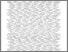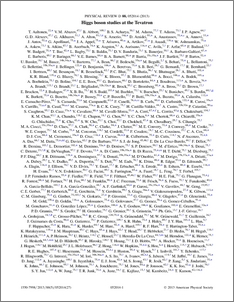# Higgs Boson Studies at the Tevatron

Collaboration, CDF and Bertram, Iain and Borissov, Guennadi and Fox, Harald and Ratoff, Peter and D0 Collaboration, The (2013) Higgs Boson Studies at the Tevatron. Physical Review D, 88 (5). ISSN 1550-7998Preview
PDF (PhysRevD.88.052014)
PhysRevD.88.052014.pdf - Published Version

We combine searches by the CDF and D0 Collaborations for the standard model Higgs boson with mass in the range 90--200 GeV$/c^2$ produced in the gluon-gluon fusion, $WH$, $ZH$, $t{\bar{t}}H$, and vector boson fusion processes, and decaying in the $H\rightarrow b{\bar{b}}$, $H\rightarrow W^+W^-$, $H\rightarrow ZZ$, $H\rightarrow\tau^+\tau^-$, and $H\rightarrow \gamma\gamma$ modes. The data correspond to integrated luminosities of up to 10 fb$^{-1}$ and were collected at the Fermilab Tevatron in $p{\bar{p}}$ collisions at $\sqrt{s}=1.96$ TeV. The searches are also interpreted in the context of fermiophobic and fourth generation models. We observe a significant excess of events in the mass range between 115 and 140 GeV/$c^2$. The local significance corresponds to 3.0 standard deviations at $m_H=125$ GeV/$c^2$, consistent with the mass of the Higgs boson observed at the LHC, and we expect a local significance of 1.9 standard deviations. We separately combine searches for $H \to b\bar{b}$, $H \to W^+W^-$, $H\rightarrow\tau^+\tau^-$, and $H\rightarrow\gamma\gamma$. The observed signal strengths in all channels are consistent with the presence of a standard model Higgs boson with a mass of 125 GeV/$c^2$.View Item